## Wednesday, May 22, 2019

### Fix to default prior and many other updates to `mcmcMk` & its methods

The phytools package has a little-used function called `mcmcMk` for doing a Bayesian MCMC analysis of the (extended) Mk model of Lewis (2001). A user contacted me about some weird results he had obtained from the function. It turns out this was due to a strange choice of default prior that I can't really explain but turned out to be much more informative than intended.

In fixing this issue I discovered that in other ways the function is quite neat, and so I have developed a bunch of different new S3 methods that I think could make it much more useful.

Here I'll demonstrate this a little bit, first using some simulated data - and then subsequently moving on to an empirical dataset for feeding mode in eels.

``````library(phytools)
packageVersion("phytools")
``````
``````##  '0.6.75'
``````

(Obviously, since this is new functionality if you are reading this shortly after it was written you may have to update phytools from GitHub to get this package version.)

Now let's inspect our data which consists of a simulated 200 taxon tree & two character vectors, `x` & `y`:

``````tree
``````
``````##
## Phylogenetic tree with 200 tips and 199 internal nodes.
##
## Tip labels:
##  t6, t103, t186, t189, t190, t56, ...
##
## Rooted; includes branch lengths.
``````
``````x
``````
``````##   t6 t103 t186 t189 t190  t56  t57  t16 t127 t128  t43  t29  t14  t25  t76
##    b    a    b    b    b    b    b    b    a    a    a    b    b    a    a
##  t77  t82  t83 t137 t138 t101  t27 t122 t123   t4  t10  t17  t94  t95  t75
##    b    a    a    b    b    b    a    b    b    a    a    b    a    a    a
## t131 t132 t104 t105  t69  t65  t66  t26 t187 t188  t34  t81 t109 t110 t180
##    a    a    a    a    a    a    a    a    a    a    a    a    a    a    a
## t181   t5   t8  t44  t45 t149 t150  t89 t169 t170 t111 t112  t61 t193 t194
##    a    a    a    a    a    a    a    a    a    a    a    a    a    a    a
##  t18   t2  t72 t113 t114 t151 t152  t32  t41  t42 t167 t168   t7  t30  t31
##    a    a    a    a    a    a    a    a    b    b    a    a    a    b    a
##  t40 t106 t107  t38  t39  t19  t67  t68  t53 t195 t196 t100 t161 t162 t159
##    b    a    a    a    a    a    a    a    a    a    a    a    a    a    a
## t160 t173 t174 t148  t87  t88  t64  t46 t165 t166  t47  t48 t139 t157 t158
##    a    a    a    a    a    a    a    a    a    b    a    b    b    b    b
## t184 t185 t175 t125  t28  t51  t52   t9  t36 t176 t177  t92  t93  t71 t197
##    a    a    a    a    b    b    b    b    b    b    b    b    b    b    b
## t198  t37  t86 t191 t192   t3 t163 t164  t20  t21 t178 t179  t35  t79  t80
##    b    b    b    b    b    a    a    a    b    a    b    b    a    a    a
##  t59  t58  t70 t129 t130  t78  t33  t54  t55 t115 t116  t12 t182 t183  t96
##    a    a    a    a    a    a    a    a    a    a    a    a    a    a    a
##  t97 t171 t172  t90  t91  t98  t99 t140 t141  t11  t13 t117 t153 t154 t126
##    a    a    a    b    a    a    a    a    a    a    a    a    a    b    a
##  t22 t146 t147  t23  t24 t124 t144 t145 t135 t136 t108  t50 t142 t143  t73
##    a    a    a    a    a    a    a    a    a    a    a    a    a    a    a
##  t74  t84  t85 t120 t121 t133 t134  t60 t155 t156 t199 t200 t102  t62  t63
##    a    a    a    a    a    a    a    a    a    a    b    b    b    a    a
##  t15 t118 t119  t49   t1
##    a    a    a    a    a
## Levels: a b
``````
``````y
``````
``````##   t6 t103 t186 t189 t190  t56  t57  t16 t127 t128  t43  t29  t14  t25  t76
##    B    A    A    A    A    A    A    A    B    B    C    A    A    B    A
##  t77  t82  t83 t137 t138 t101  t27 t122 t123   t4  t10  t17  t94  t95  t75
##    A    A    A    A    A    A    A    C    C    A    A    B    B    B    B
## t131 t132 t104 t105  t69  t65  t66  t26 t187 t188  t34  t81 t109 t110 t180
##    B    B    A    A    A    A    A    A    A    A    A    A    A    A    A
## t181   t5   t8  t44  t45 t149 t150  t89 t169 t170 t111 t112  t61 t193 t194
##    A    A    A    B    B    B    B    B    B    B    B    B    B    A    A
##  t18   t2  t72 t113 t114 t151 t152  t32  t41  t42 t167 t168   t7  t30  t31
##    B    A    A    A    A    A    A    A    A    A    A    A    A    A    A
##  t40 t106 t107  t38  t39  t19  t67  t68  t53 t195 t196 t100 t161 t162 t159
##    B    A    A    A    A    B    A    A    B    A    A    B    A    A    C
## t160 t173 t174 t148  t87  t88  t64  t46 t165 t166  t47  t48 t139 t157 t158
##    C    C    C    C    B    A    B    B    B    B    C    B    C    C    C
## t184 t185 t175 t125  t28  t51  t52   t9  t36 t176 t177  t92  t93  t71 t197
##    A    A    A    B    B    B    C    B    B    B    B    B    C    A    B
## t198  t37  t86 t191 t192   t3 t163 t164  t20  t21 t178 t179  t35  t79  t80
##    B    B    B    B    B    B    A    A    A    C    A    A    A    A    A
##  t59  t58  t70 t129 t130  t78  t33  t54  t55 t115 t116  t12 t182 t183  t96
##    A    A    A    A    A    A    A    A    A    A    A    A    A    A    A
##  t97 t171 t172  t90  t91  t98  t99 t140 t141  t11  t13 t117 t153 t154 t126
##    A    A    A    A    A    A    A    A    A    A    A    A    A    A    A
##  t22 t146 t147  t23  t24 t124 t144 t145 t135 t136 t108  t50 t142 t143  t73
##    A    A    A    A    A    A    A    A    A    A    A    A    A    A    A
##  t74  t84  t85 t120 t121 t133 t134  t60 t155 t156 t199 t200 t102  t62  t63
##    A    A    A    A    A    A    A    B    A    A    B    A    A    A    A
##  t15 t118 t119  t49   t1
##    A    A    A    A    C
## Levels: A B C
``````

Now we can proceed to run our MCMC for the first character vector `x`. We can assume any of the standard extended Mk models, but here I will set `model="ARD"`:

``````mcmc.x<-mcmcMk(tree,x,model="ARD",prop.var=0.005,
ngen=10000,print=500)
``````
``````## Running MCMC....
## gen  [b,a]   [a,b]   logLik
## 1    0.0492  0.0492  -107.356
## 501  0.8713  1.1106  -78.4027
## 1001 1.5521  0.9809  -75.4808
## 1501 1.3616  0.9887  -75.8263
## 2001 1.6947  0.9337  -74.9609
## 2501 1.6446  0.7872  -74.0923
## 3001 1.205   0.9015  -75.3249
## 3501 3.1329  0.9437  -75.7518
## 4001 1.9646  0.4388  -74.3684
## 4501 3.7514  1.0544  -77.2086
## 5001 4.9081  1.248   -80.1114
## 5501 3.8791  0.9513  -77.2952
## 6001 3.6613  0.9009  -76.7296
## 6501 2.9479  0.3835  -76.8355
## 7001 1.3162  0.8561  -74.8016
## 7501 1.6166  0.6805  -73.7287
## 8001 0.7741  0.6074  -74.6178
## 8501 0.9214  0.3527  -75.8891
## 9001 1.6804  0.386   -74.73
## 9501 1.6623  0.8166  -74.2324
## Done.
``````
``````print(mcmc.x)
``````
``````##
## Posterior sample from mcmcMk consisting of a posterior sample obtained using
## Bayesian MCMC in the form of a matrix.
##
## 1. plot('object_name') will create a likelihood profile plot.
## 2. summary('object_name') will compute a summary of the MCMC.
## 3. density('object_name') will calculate a posterior density from the sample.
## 4. Finally, plot(density('object_name')) will plot the posterior density and
##    and high probability density intervals.
##
## To work best, we recommend users install the package 'coda'.
``````

The S3 `plot` method gives us a likelihood profile for our MCMC. Let's try it:

``````plot(mcmc.x)
``````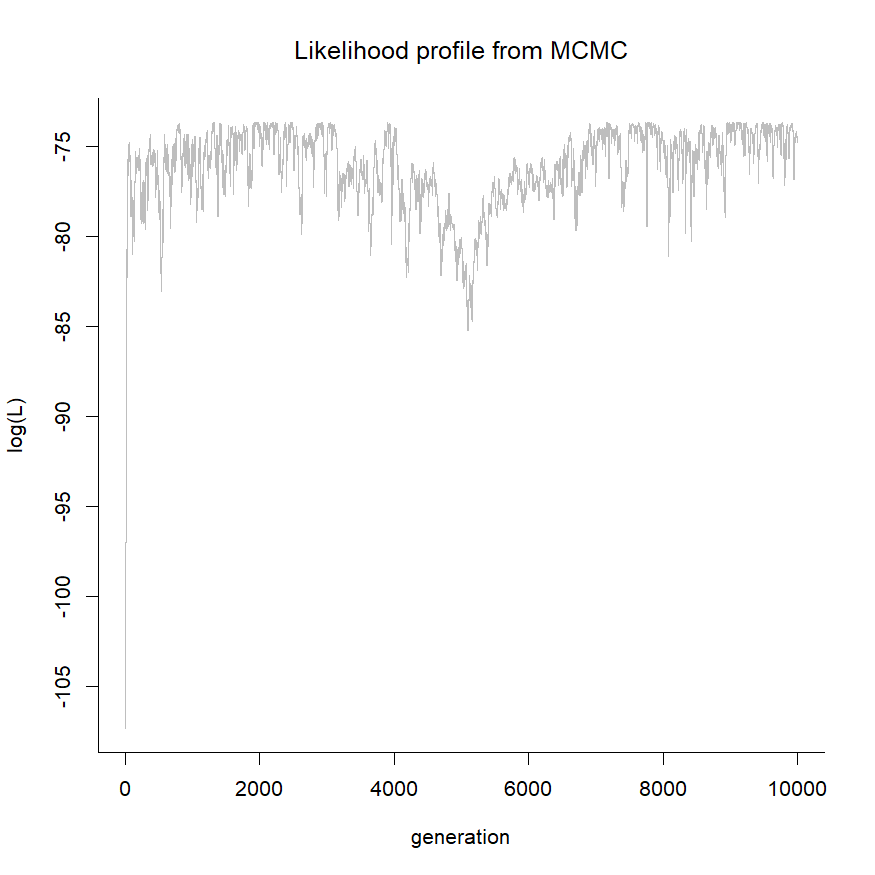It's a bit hard to tell if this is well-mixed or not, but let's just assume that it is!

The S3 `summary` method gives (unsurprisingly) a summary of our posterior sample - in particularly an average value of the transition matrix Q and HPD intervals around each of the transition rates between states:

``````summary(mcmc.x)
``````
``````## Assuming 20% burn-in as no burn-in was specified....
##
## Mean value of Q from the post burn-in posterior sample:
##            a          b
## a -0.8434544  0.8434544
## b  2.4166152 -2.4166152
##
## 95% HPD interval computed either from the post burn-in
## samples or using 'coda':
##           lower    upper
## [b,a] 0.6779169 4.591337
## [a,b] 0.2849471 1.553939
``````

Note (as indicated) that the method discards the first 20% of our generations of MCMC as burn-in unless we tell it otherwise, which we can do, of course:

``````summary(mcmc.x,burnin=5000)
``````
``````## Mean value of Q from the post burn-in posterior sample:
##            a          b
## a -0.8293531  0.8293531
## b  2.2536256 -2.2536256
##
## 95% HPD interval computed either from the post burn-in
## samples or using 'coda':
##           lower    upper
## [b,a] 0.6173297 4.345430
## [a,b] 0.2849471 1.631915
``````

I also added a `density` and associated `plot` function as follows:

``````pd.x<-density(mcmc.x,bw=0.1)
``````
``````## Assuming 20% burn-in as no burn-in was specified....
``````
``````print(pd.x)
``````
``````##
##  Density [b,a]
##
##        x                y
##  Min.   :0.1327   Min.   :0.0000277
##  1st Qu.:1.4867   1st Qu.:0.0855801
##  Median :2.8407   Median :0.1548434
##  Mean   :2.8407   Mean   :0.1844559
##  3rd Qu.:4.1947   3rd Qu.:0.2933771
##  Max.   :5.5487   Max.   :0.4307307
##
##  Density [b,a]
##
##        x                y
##  Min.   :0.1327   Min.   :0.0000277
##  1st Qu.:1.4867   1st Qu.:0.0855801
##  Median :2.8407   Median :0.1548434
##  Mean   :2.8407   Mean   :0.1844559
##  3rd Qu.:4.1947   3rd Qu.:0.2933771
##  Max.   :5.5487   Max.   :0.4307307
##
## To plot enter plot('object_name') at the command line interface.
``````

But the `plot` method for this custom density object class is definitely cooler. Let's check it out:

``````plot(pd.x)
``````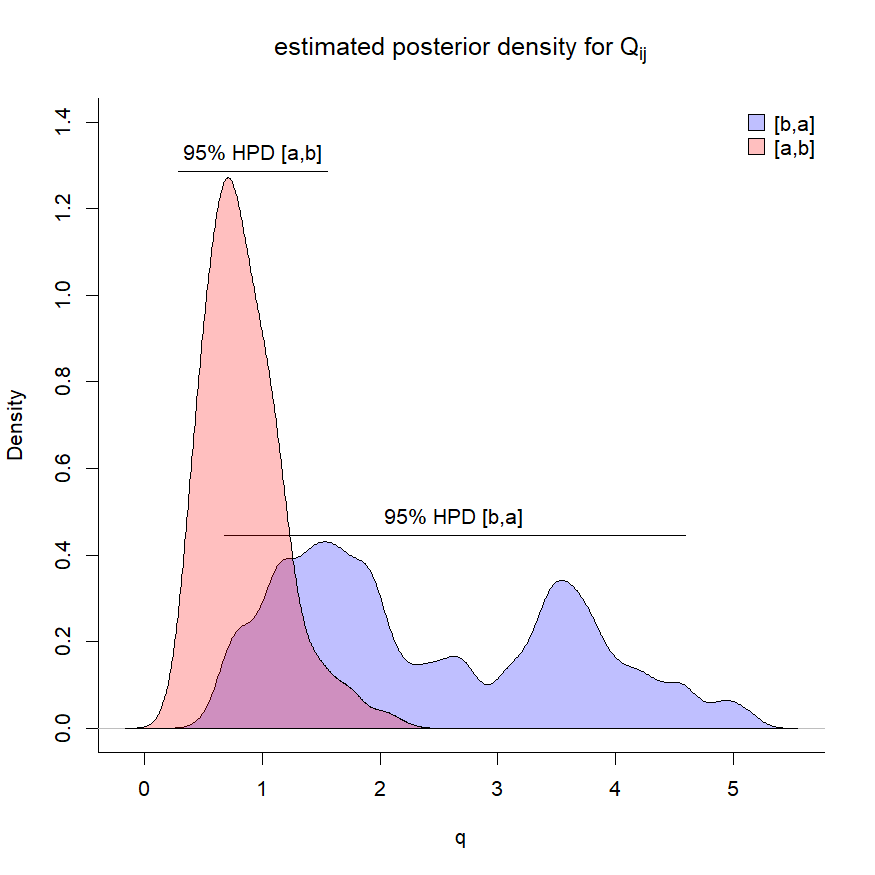Neat. Now, let's do something similar for `y` which contains a three-state character. In this case, I happen to know that the data were simulated under an ordered process in which transitions are only permitted between adjacent states.

Let's impose that model on our Bayesian inference. I'm also going to adjust the variance of the normal proposal distribution. By default this would be 0.01/total tree length (in this case, also 0.01), but here I'm going to set it to 0.05:

``````model<-matrix(c(
0,1,0,
2,0,3,
0,4,0),3,3,byrow=TRUE,
dimnames=list(levels(y),levels(y)))
model
``````
``````##   A B C
## A 0 1 0
## B 2 0 3
## C 0 4 0
``````
``````mcmc.y<-mcmcMk(tree,y,model=model,prop.var=0.05,
ngen=20000,print=500)
``````
``````## Running MCMC....
## gen  [A,B]   [B,A]   [B,C]   [C,B]   logLik
## 1    0.0738  0.0738  0.0738  0.0738  -139.9116
## 501  0.7929  2.3011  1.2086  3.0696  -100.0168
## 1001 0.2424  1.9118  1.968   1.3895  -106.8495
## 1501 0.8968  0.8617  1.2571  1.4952  -100.8796
## 2001 0.9987  3.5494  0.4307  2.5344  -103.6955
## 2501 0.3571  0.9037  1.4929  3.1541  -101.0945
## 3001 0.527   1.8505  0.8831  1.6118  -99.9354
## 3501 0.9003  2.9273  3.3041  2.5723  -107.8218
## 4001 0.303   3.7478  0.8807  6.2265  -105.8216
## 4501 0.4373  2.6688  2.6178  5.7192  -102.596
## 5001 0.6424  1.5677  0.5674  7.6649  -104.0805
## 5501 0.9437  4.3249  1.5454  9.1119  -108.0044
## 6001 0.3885  5.0376  2.8744  10.2261 -112.6765
## 6501 2.1845  4.8637  3.6409  9.5295  -116.2657
## 7001 1.4617  4.7083  4.1376  8.6136  -111.5319
## 7501 0.4512  1.653   3.5337  12.5136 -103.1212
## 8001 1.1108  2.6291  5.2321  11.1377 -107.3971
## 8501 0.4506  0.6254  5.3749  11.2217 -105.4739
## 9001 0.669   0.9857  1.4964  9.7234  -102.6163
## 9501 0.5219  1.6389  2.2034  8.0083  -100.9076
## 10001    0.591   1.6407  1.3879  8.3047  -101.3362
## 10501    1.3114  2.2078  1.5595  7.6294  -105.3352
## 11001    1.3235  2.3554  1.6809  8.5757  -105.9546
## 11501    0.5321  0.6261  2.9792  11.9312 -103.3113
## 12001    0.5009  2.491   1.2613  8.5838  -102.7391
## 12501    0.8139  5.936   0.6449  1.6821  -111.3256
## 13001    0.565   0.2615  1.5711  0.6184  -102.4311
## 13501    0.7858  2.3639  0.3216  2.8565  -101.902
## 14001    0.9089  1.315   1.5638  1.41    -101.2064
## 14501    0.6014  1.5487  2.2075  3.0507  -100.8305
## 15001    0.751   0.3838  1.5209  2.6174  -101.5443
## 15501    0.2071  2.9983  0.6016  3.4198  -102.2142
## 16001    0.3475  1.6659  1.9359  5.5227  -100.872
## 16501    0.6605  1.7695  1.6263  9.6205  -102.0407
## 17001    0.6212  0.8155  1.1363  6.6569  -101.3515
## 17501    0.471   2.7336  3.3019  5.6302  -104.1388
## 18001    0.4604  2.6996  4.9081  5.4362  -108.5106
## 18501    0.6036  1.4582  4.7579  4.6932  -106.5785
## 19001    0.193   3.2469  4.4128  6.0448  -110.3741
## 19501    1.0267  1.7573  4.7954  5.2047  -108.3454
## Done.
``````
``````mcmc.y
``````
``````##
## Posterior sample from mcmcMk consisting of a posterior sample obtained using
## Bayesian MCMC in the form of a matrix.
##
## 1. plot('object_name') will create a likelihood profile plot.
## 2. summary('object_name') will compute a summary of the MCMC.
## 3. density('object_name') will calculate a posterior density from the sample.
## 4. Finally, plot(density('object_name')) will plot the posterior density and
##    and high probability density intervals.
##
## To work best, we recommend users install the package 'coda'.
``````
``````plot(mcmc.y)
``````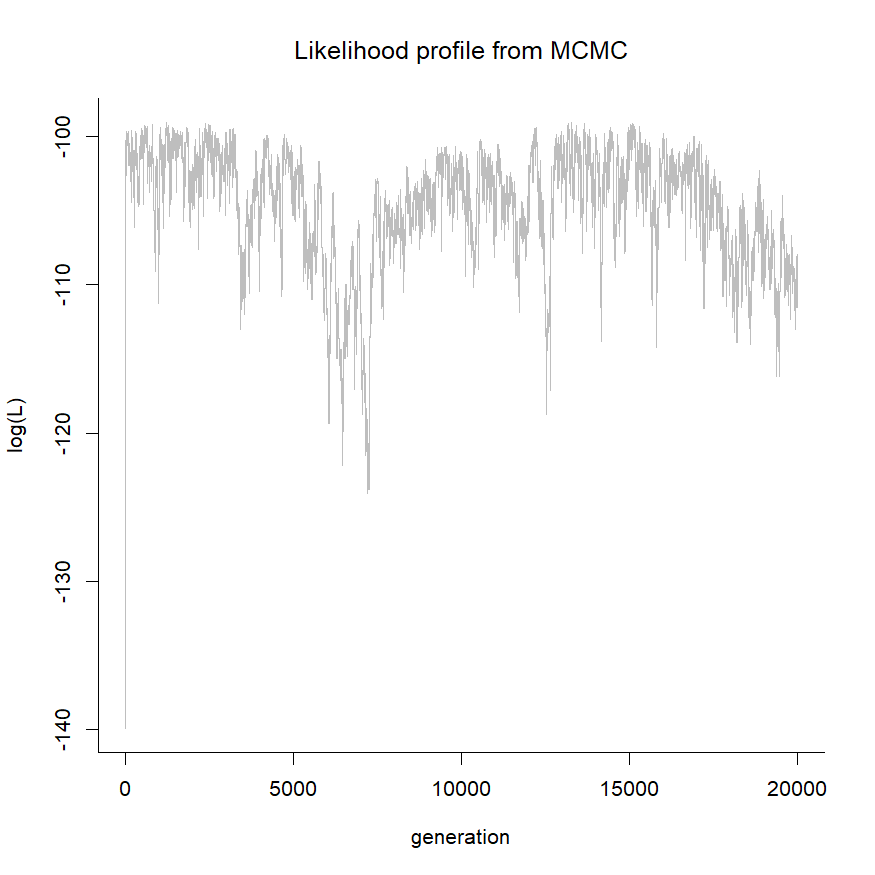``````summary(mcmc.y,burnin=5000)
``````
``````## Mean value of Q from the post burn-in posterior sample:
##            A          B         C
## A -0.8078591  0.8078591  0.000000
## B  2.3106788 -4.8984632  2.587784
## C  0.0000000  6.6205242 -6.620524
##
## 95% HPD interval computed either from the post burn-in
## samples or using 'coda':
##           lower     upper
## [A,B] 0.1839739  1.680220
## [B,A] 0.2294231  5.016908
## [B,C] 0.2186470  5.469995
## [C,B] 1.0242727 12.421114
``````
``````pd.y<-density(mcmc.y,burnin=5000)
plot(pd.y)
``````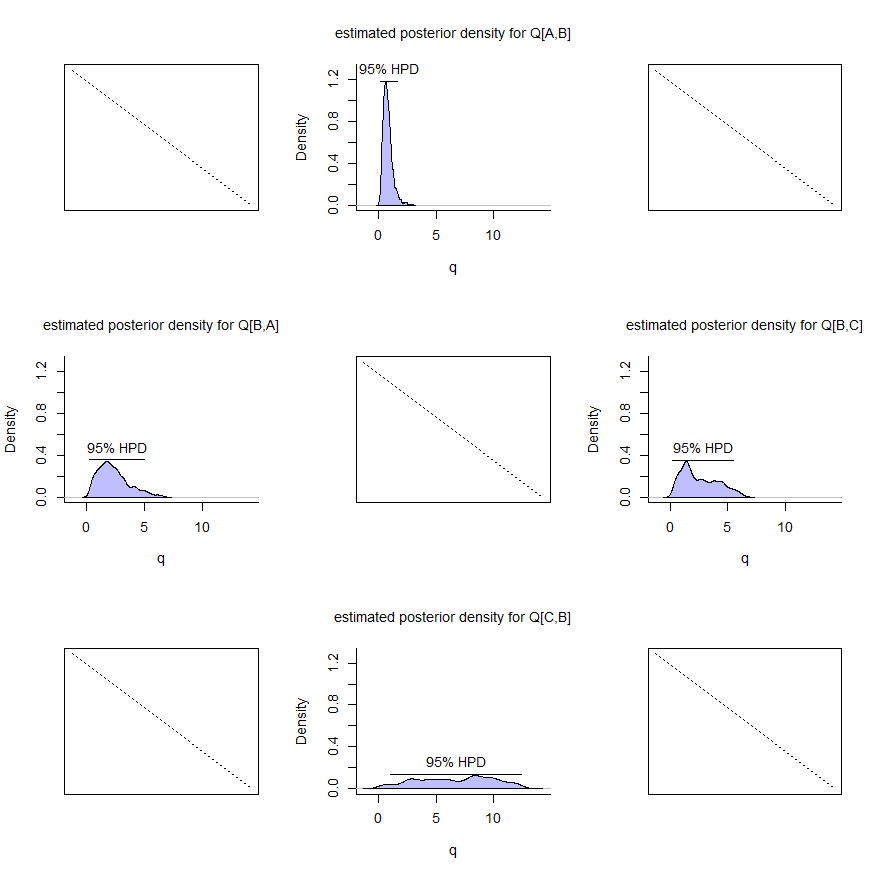Probably convergence looks kind of bad here. If so, we might try to adjust our proposal distributions (which we can do independently for each variable in the model) to get rejection rates around 75%. We can easily compute rejection rates, but when doing so we need to remember that only one variable is updated for each generation so we should first thin our posterior sample accordingly:

``````mcmc.thinned<-mcmc.y[seq(5001,20000,by=4),]
library(coda)
rejectionRate(as.mcmc(mcmc.thinned))
``````
``````##        gen      [A,B]      [B,A]      [B,C]      [C,B]     logLik
## 0.00000000 0.25313417 0.29394505 0.09975994 0.10962923 0.09975994
``````

Pretty cool. Finally, as promised we can try this out with some empirical data for elopomorph eels and feeding mode. Luke Harmon & I have used these data in a bunch of different workshops, but they were originally kindly provided to me by Dave Collar.

``````eel.tree<-read.tree(file="http://www.phytools.org/TS2018/data/elopomorph.tre")
row.names=1)
plotTree(eel.tree,ftype="i",fsize=0.7)
``````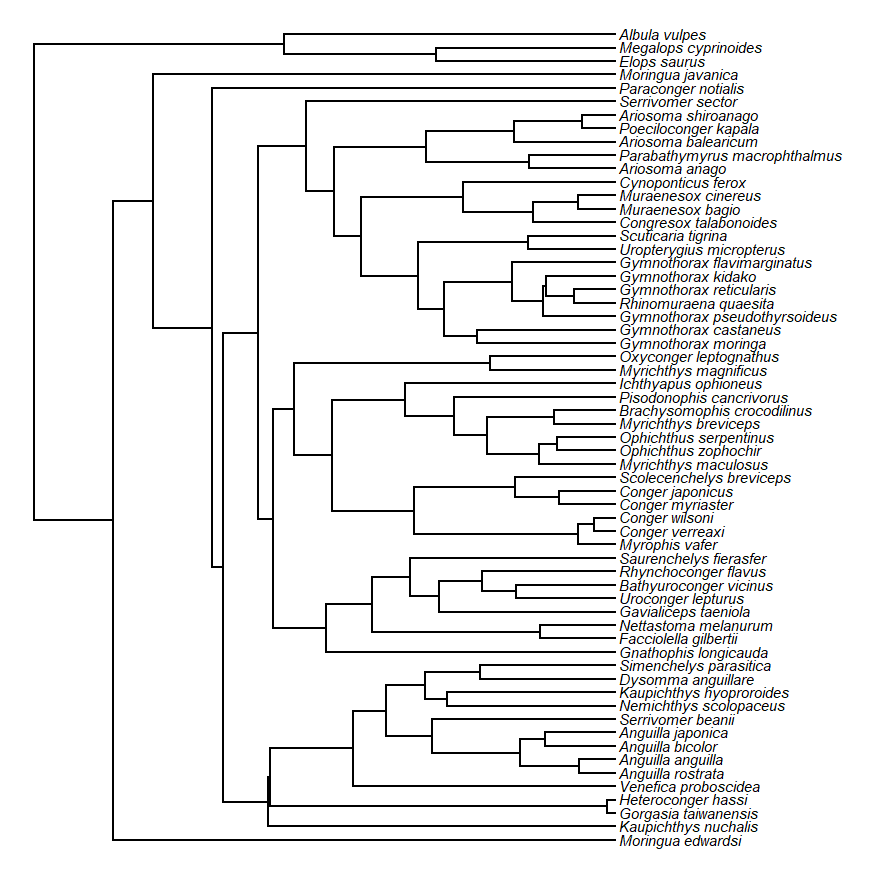``````head(eel.data)
``````
``````##                   feed_mode Max_TL_cm
## Albula_vulpes       suction       104
## Anguilla_anguilla   suction        50
## Anguilla_bicolor    suction       120
## Anguilla_japonica   suction       150
## Anguilla_rostrata   suction       152
## Ariosoma_anago      suction        60
``````
``````fmode<-setNames(eel.data\$feed_mode,rownames(eel.data))
``````

It turns out the `"ER"` model fits these data pretty well - so let's just assume that from the get-go:

``````mcmc.fmode<-mcmcMk(eel.tree,fmode,model="ER",
ngen=10000,print=500)
``````
``````## Running MCMC....
## gen  [suction,bite]  logLik
## 1    0.001   -59.2835
## 501  0.038   -38.8745
## 1001 0.0109  -37.4891
## 1501 0.101   -41.3336
## 2001 0.056   -40.1027
## 2501 0.0158  -37.0331
## 3001 0.0184  -37.0997
## 3501 0.0268  -37.7966
## 4001 0.0118  -37.3078
## 4501 0.0167  -37.0422
## 5001 0.0212  -37.2865
## 5501 0.0286  -37.9776
## 6001 0.0196  -37.1705
## 6501 0.0153  -37.0366
## 7001 0.0155  -37.0348
## 7501 0.0117  -37.3271
## 8001 0.0241  -37.5334
## 8501 0.0215  -37.311
## 9001 0.0169  -37.0475
## 9501 0.0166  -37.0406
## Done.
``````
``````plot(mcmc.fmode)
``````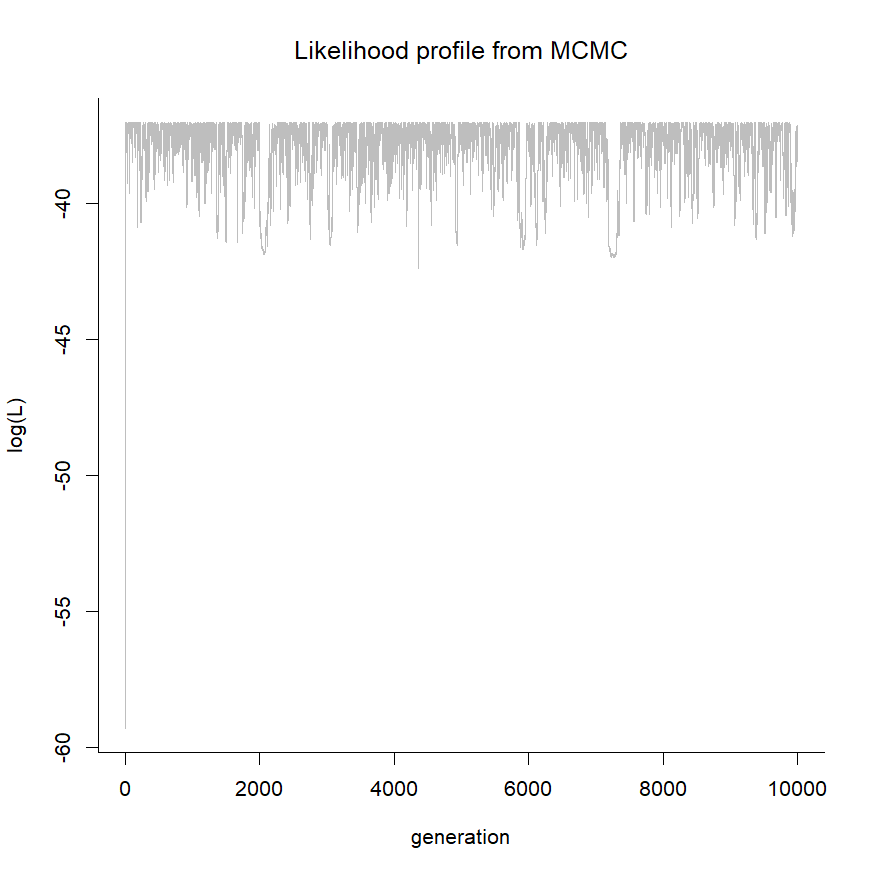``````summary(mcmc.fmode)
``````
``````## Assuming 20% burn-in as no burn-in was specified....
##
## Mean value of Q from the post burn-in posterior sample:
##                bite     suction
## bite    -0.03000165  0.03000165
## suction  0.03000165 -0.03000165
##
## 95% HPD interval computed either from the post burn-in
## samples or using 'coda':
##                      lower      upper
## [suction,bite] 0.005749017 0.08181328
``````
``````pd.fmode<-density(mcmc.fmode,bw=0.002)
``````
``````## Assuming 20% burn-in as no burn-in was specified....
``````
``````plot(pd.fmode)
``````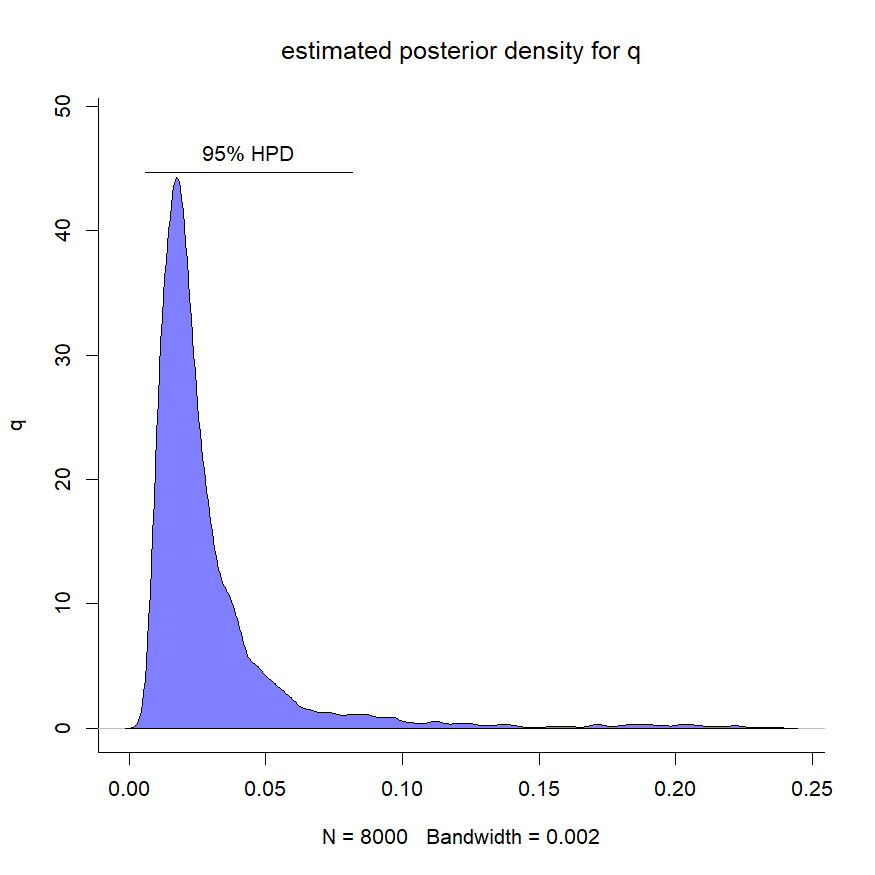One thing you might notice is that the posterior distribution for q is quite asymmetric. This (predictably) means that our average from the posterior sample may not be the best way to summarize the posterior distribution. It will also tend to cause the mean from the posterior sample to be higher than our MLEs. For instance:

``````fitMk(eel.tree,fmode,model="ER")
``````
``````## Object of class "fitMk".
##
## Fitted (or set) value of Q:
##              bite   suction
## bite    -0.015828  0.015828
## suction  0.015828 -0.015828
##
## Fitted (or set) value of pi:
##    bite suction
##     0.5     0.5
##
## Log-likelihood: -37.033072
##
## Optimization method used was "nlminb"
``````

This is not an error. In fact, if we compare out likelihood surface for q to the posterior distribution we should see that they match quite closely:

``````q<-seq(0.001,0.2,by=0.001)
lik<-sapply(q,function(q,t,x) logLik(fitMk(t,x,
fixedQ=matrix(c(-q,q,q,-q),2,2))),
t=eel.tree,x=fmode)
par(mfrow=c(2,1),mar=c(4.1,4.1,3.1,1.1))
plot(q,exp(lik),type="l",ylab="likelihood",xlab="q",
bty="l",col="blue",lwd=2,main="likelihood surface",
font.main=1,xlim=c(0,0.2))
plot(pd.fmode,xlim=c(0,0.2))
``````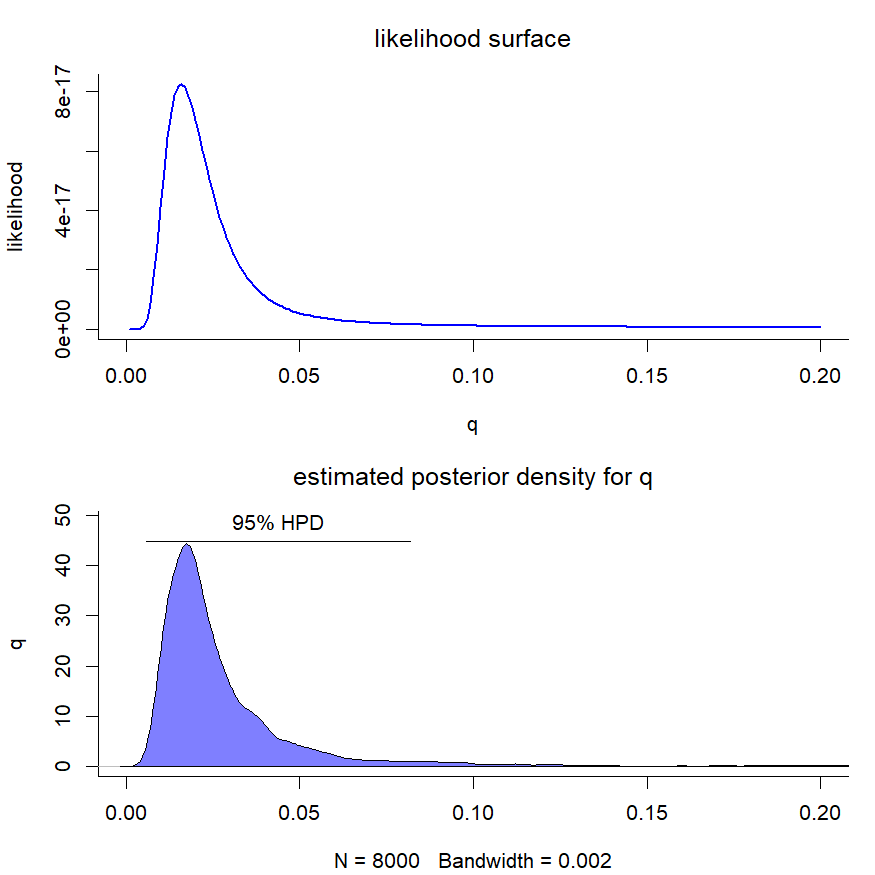which is what we'd expect if our prior probability density is fairly uninformative. (Though it's not an error, it might suggest that summarizing the posterior sample with its mean could be misleading.)

For the reader's reference, my simulated data were generated as follows:

``````set.seed(1001)
tree<-pbtree(n=200,scale=1)
Q<-matrix(c(
-0.5,0.5,
2,-2),2,2,byrow=TRUE,
dimnames=list(letters[1:2],letters[1:2]))
x<-sim.Mk(tree,Q)

Q<-matrix(c(
-0.5,0.5,0,
2,-2.5,0.5,
0,2,-2),3,3,byrow=TRUE,
dimnames=list(LETTERS[1:3],
LETTERS[1:3]))
y<-sim.Mk(tree,Q)
``````

That's all for now!